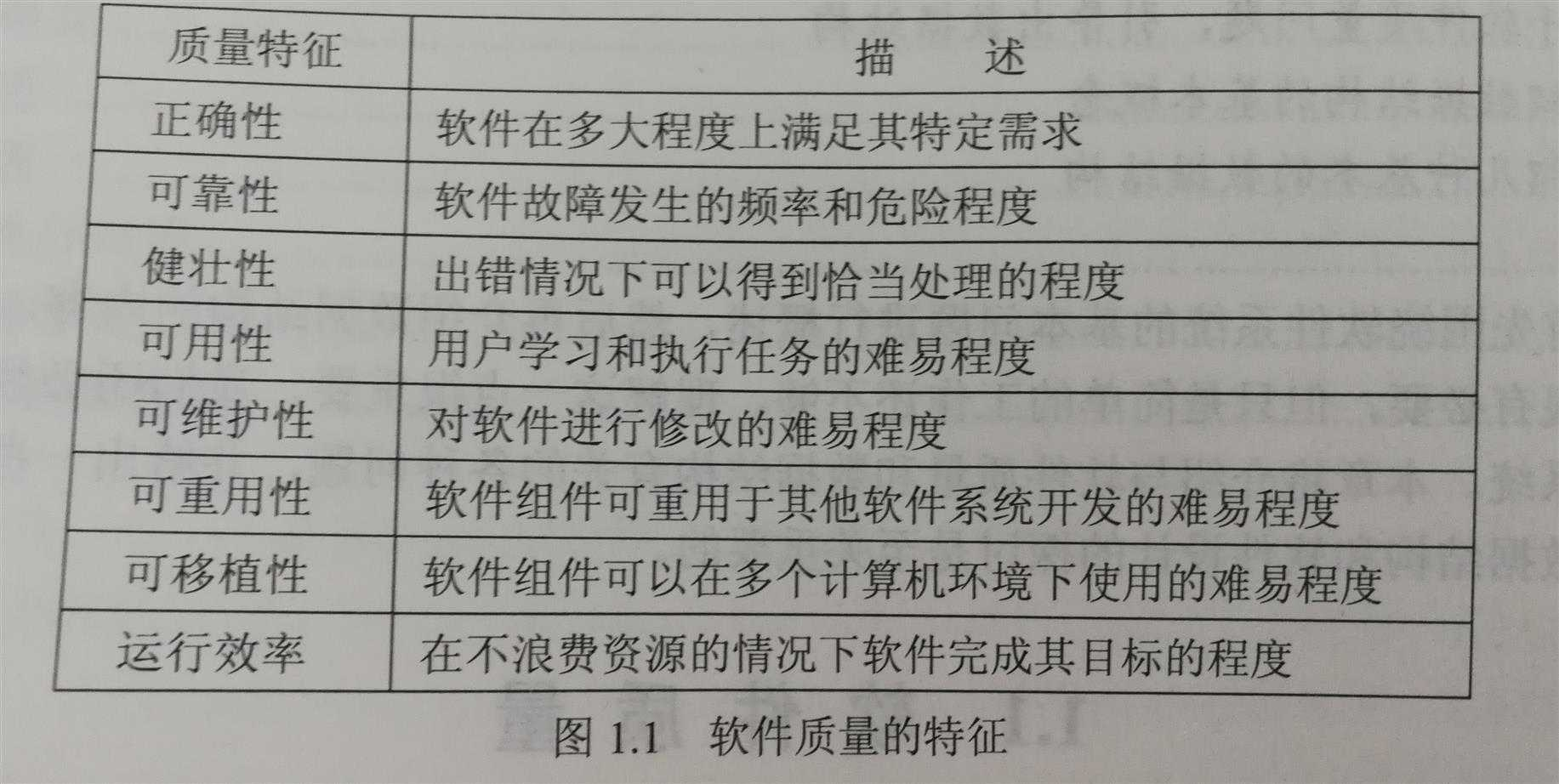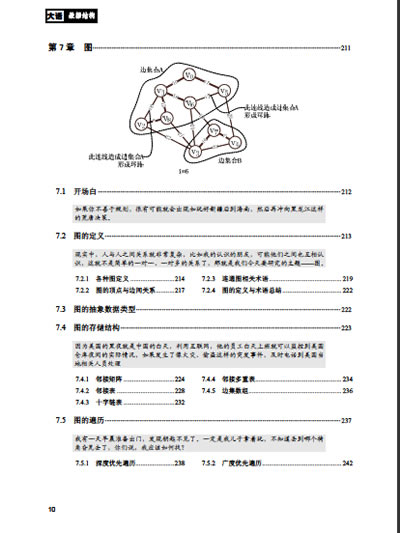# 使用数据结构给女朋友写个Html5走迷宫游戏_html5教程技巧

1.女朋百友的永远是对的。2.自己永远是错的。3.女朋友是度拿来宠的，不是吵架的。4.女朋友永问远是第一顺位。5.女朋友永答远是最美的。内6.女朋友是最温柔的。7.自己永远最爱女朋友。抱歉，我不是男人，找容不到那么多理由www.zgxue.com防采集请勿采集本网。<!DOCTYPE html><html> <head> <title>MyHtml.html</title> </head> <body> <canvas id="mycanvas" width="600px" height="600px"></canvas> </body> <script type="text/javascript">var aa=14; var chess = document.getElementById("mycanvas"); var context = chess.getContext('2d'); // var context2 = chess.getContext('2d'); // context.strokeStyle = 'yellow'; var tree = [];//存放是否联通 var isling=[];//判断是否相连 for(var i=0;i<aa;i++){ tree[i]=[]; for(var j=0;j<aa;j++){ tree[i][j]=-1;//初始值为0 } } for(var i=0;i<aa*aa;i++){ isling[i]=[]; for(var j=0;j<aa*aa;j++){ isling[i][j]=-1;//初始值为0 } } function drawChessBoard(){//绘画 for(var i=0;i<aa+1;i++){ context.strokeStyle='gray';//可选区域 context.moveTo(15+i*30,15);//垂直方向画15根线，相距30px; context.lineTo(15+i*30,15+30*aa); context.stroke(); context.moveTo(15,15+i*30);//水平方向画15根线，相距30px;棋盘为14*14； context.lineTo(15+30*aa,15+i*30); context.stroke(); } } drawChessBoard();//绘制棋盘 // var mymap=new Array(36); // for(var i=0;i<36;i++) // {mymap[i]=-1;} </script></html>`我们的随机迷宫`：在每个方格都不联通的情况下，是一个棋盘方格，这也是它的初始状态。而这个节点可以跟邻居可能相连，也可能不相连。我们可以通过`并查集`实现。

1：定义好不想交集合的基本类和方法（`search,union`等）

2：数组初始化，每一个数组元素都是一个集合，值为-1

3：随机查找一个格子（一维数据要转换成二维，有点麻烦），在随机找一面墙（也就是找这个格子的上下左右），还要判断找的格子出没出界。

4：判断两个格子(一维数组编号)是否在一个集合(并查集查找)。如果在，则重新找，如果不在，那么把墙挖去

5：把墙挖去有点繁琐，需要考虑奇偶判断它那种墙(上下还是左右，还要考虑位置)，然后擦掉。（根据数组转换成真实距离）。具体为找一个节点，根据位置关系找到一维数组的号位用并查集判断是否在一个集合中。

6：最终得到一个完整的迷宫。直到第一个(1,1)和(n,n)联通停止。虽然采用随机数找墙，但是效果并不是特别差。其中要搞清一维二维数组的关系。一维是真实数据，并查集操作。二维是位置。要搞懂转化！while(search(0)!=search(aa*aa-1))//主要思路 { var num = parseInt(Math.random() * aa*aa );//产生一个小于196的随机数 var neihbour=getnei(num); if(search(num)==search(neihbour)){continue;} else//不在一个上 { isling[num][neihbour]=1;isling[neihbour][num]=1; drawline(num,neihbour);//划线 union(num,neihbour); } }

<!DOCTYPE html><html> <head> <title>MyHtml.html</title> </head> <body> <canvas id="mycanvas" width="600px" height="600px"></canvas> </body> <script type="text/javascript">//自行添加上面代码 // var mymap=new Array(36); // for(var i=0;i<36;i++) // {mymap[i]=-1;} function getnei(a)//获得邻居号 random { var x=parseInt(a/aa);//要精确成整数 var y=a%aa; var mynei=new Array();//储存邻居 if(x-1>=0){mynei.push((x-1)*aa+y);}//上节点 if(x+1<14){mynei.push((x+1)*aa+y);}//下节点 if(y+1<14){mynei.push(x*aa+y+1);}//有节点 if(y-1>=0){mynei.push(x*aa+y-1);}//下节点 var ran=parseInt(Math.random() * mynei.length ); return mynei[ran]; } function search(a)//找到根节点 { if(tree[parseInt(a/aa)][a%aa]>0)//说明是子节点 { return search(tree[parseInt(a/aa)][a%aa]);//不能压缩路径路径压缩 } else return a; } function value(a)//找到树的大小 { if(tree[parseInt(a/aa)][a%aa]>0)//说明是子节点 { return tree[parseInt(a/aa)][a%aa]=value(tree[parseInt(a/aa)][a%aa]);//不能路径压缩 } else return -tree[parseInt(a/aa)][a%aa]; } function union(a,b)//合并 { var a1=search(a);//a根 var b1=search(b);//b根 if(a1==b1){} else { if(tree[parseInt(a1/aa)][a1%aa]<tree[parseInt(b1/aa)][b1%aa])//这个是负数()，为了简单减少计算，不在调用value函数 { tree[parseInt(a1/aa)][a1%aa]+=tree[parseInt(b1/aa)][b1%aa];//个数相加 注意是负数相加 tree[parseInt(b1/aa)][b1%aa]=a1; //b树成为a树的子树，b的根b1直接指向a； } else { tree[parseInt(b1/aa)][b1%aa]+=tree[parseInt(a1/aa)][a1%aa]; tree[parseInt(a1/aa)][a1%aa]=b1;//a所在树成为b所在树的子树 } } } function drawline(a,b)//划线，要判断是上下还是左右 { var x1=parseInt(a/aa); var y1=a%aa; var x2=parseInt(b/aa); var y2=b%aa; var x3=(x1+x2)/2; var y3=(y1+y2)/2; if(x1-x2==1||x1-x2==-1)//左右方向的点 需要上下划线 { //alert(x1); // context.beginPath(); context.strokeStyle = 'white'; // context.moveTo(30+x3*30,y3*30+15);// // context.lineTo(30+x3*30,y3*30+45); context.clearRect(29+x3*30, y3*30+16,2,28); // context.stroke(); } else { // context.beginPath(); context.strokeStyle = 'white'; // context.moveTo(x3*30+15,30+y3*30);// // context.lineTo(45+x3*30,30+y3*30); context.clearRect(x3*30+16, 29+y3*30,28,2); // context.stroke(); } } while(search(0)!=search(aa*aa-1))//主要思路 { var num = parseInt(Math.random() * aa*aa );//产生一个小于196的随机数 var neihbour=getnei(num); if(search(num)==search(neihbour)){continue;} else//不在一个上 { isling[num][neihbour]=1;isling[neihbour][num]=1; drawline(num,neihbour);//划线 union(num,neihbour); } } </script></html>• 本文相关：
• html5实现的熊猫弹跳小游戏源码
• html5实现的卡牌配对小游戏源码
• js实现的魔塔闯关类小游戏源码
• html5 迷宫游戏(碰撞检测)实例一
• JAVA数据结构的作业，我是学网络的，女朋友要我写，悲剧啊，求帮助，感激啊~~
• 写给女朋友的100条
• 怎么给女朋友写情书
• 女朋友给了个便签，写什么好？
• 给女朋友写的作文
• 和自己的女朋友恋爱了100天，想在说说上写下一段话该怎么写啊！
• 给女朋友的信怎样写
• 写给“女朋友”的诗句有哪些？
• 写给女朋友的歌词
• 写给女朋友的情诗
• 免责声明 - 关于我们 - 联系我们 - 广告联系 - 友情链接 - 帮助中心 - 频道导航